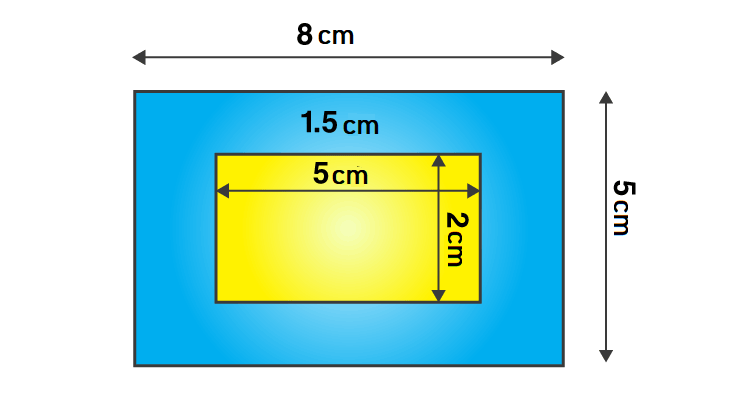Question

# A picture is painted on a cardboard $8 cm$ long and $5 cm$ wide such that there is a margin of $1.5 cm$ along each of its sides. Find the total area of the margin.v

Open in App
Solution

## GivenThe length of the cardboard $=8cm$The breadth of the cardboard$=5cm$Step 1- Area of CardboardArea of the cardboard = length × breadth $=8×5\phantom{\rule{0ex}{0ex}}=40{cm}^{2}$ Step 2- Area of cardboard when margins are not included From the figure,The new length of the cardboard when the margin is not included $=\left(8-1.5-1.5\right)cm=5cm$The new breadth of the cardboard when the margin is not included $=\left(5-1.5-1.5\right)cm=2cm$The new area of the cardboard $=$new length $×$new breadth $=5×2\phantom{\rule{0ex}{0ex}}=10{cm}^{2}$Step 3 - Area of the margin The area of margin$=$ Area of the cardboard $-$Area of the cardboard when the margin is not included $=\left(40-10\right)c{m}^{2}\phantom{\rule{0ex}{0ex}}=30{cm}^{2}$Hence, the total area of the margin is $30{cm}^{2}$Suggest Corrections0Similar questions